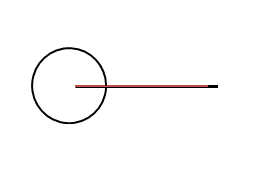Angles - Geometry
26-06-2020    62 times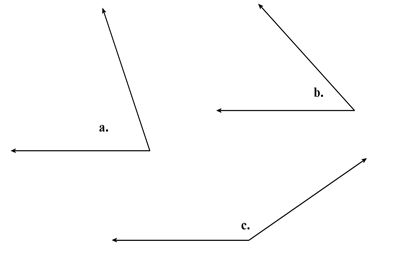When two line or line segment are intersect in such a point. Then this point is known as vertex. The amount of rotation of one line or line segment corresponding to other is called an angle θ. Angles are usually measured in degree (denoted °) or radian (denoted rad, or without a unit).

There are six types of angles are possible. Angles are divided according to their measured in degree –

1. Acute angles: Size of the angles are 0° - below 90°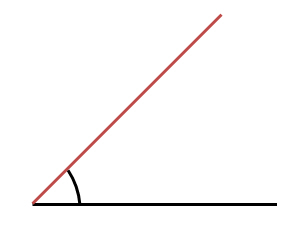2. Right angle: Size of the angles are 90°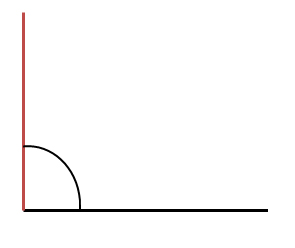3. Obtuse angle: Size of the angles are 91° - below 180°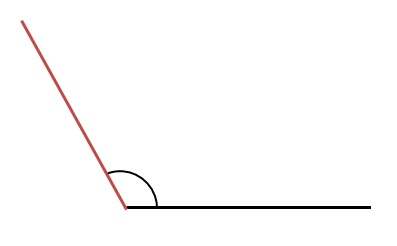4. Straight angle: Size of the angles are 180°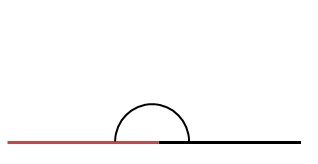5. Reflex angle: Size of the angles are 181° - below 360°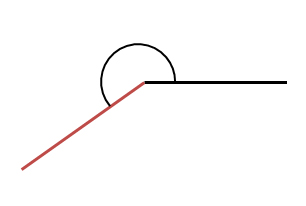6. Full angle: Size of the angles are 360°